Physics Lesson Plan on Force

# Physics Lesson Plan on Force

## Physics Lesson Plan on Force for Science Teachers

Brief Overview of the Physics Lesson Plan
• Grade – 5, 6, 7, 8 and 9
• Subject – Science (Physics)
• Topic – Force

Note: The Science Lesson Plan given below is just an example. You can change the name, class, course, date, duration, etc. according to your needs.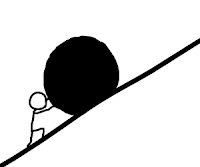General Aims  –
1. To provide the opportunity to develop scientific skills in students.
2. To develop the power of thinking and reasoning among them.
3. To develop a scientific attitude and observation among the students.
4. To develop scientific creativity in the students.

Behavioral Objectives -

• Students will be able to learn about force.
• They will also be acquainted with the types of force.

Teaching Aids –

• General Teaching Aids – Chalk, Chalk Board, Duster, Pointer
• Instructional teaching aids – Chart showing types of force

Previous knowledge assumed –

Students are expected to have knowledge about moving objects.

Previous Knowledge Testing –

In order to test the previous knowledge of the students, the teacher will ask the following questions:

 Teacher/ Trainee Teacher Questions Student’s answers What will you do to move or roll a ball?Force What do you understand by the term “Push” and “Pull”? Push - exert force in order to move Pull – to draw or tug at with force. What will you apply if you want to lift a heavy box? Pull apply What is a force? No response

The announcement of the Topic –

Finding students unable to answer the last question, the teacher/ pupil-teacher will announce the topic by saying, Well Students “today we shall study about Force”.

Presentation –

Now the teacher will present the topic to the students by using an inductive and deductive approach.

 Teaching Point Teacher activity Student activity Chalkboard work Definition of Force Question - What is force? (teacher will put a question) Answer – A push or pull acting on a body that tends to change its state of rest or motion is called force. ( after putting question teacher himself will answer the question) students will listen carefully Direction of Force Question - What is the direction of force?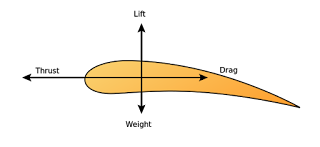The direction in which the body is pulled or pushed is called the direction of a force. Force due to an interaction Question - How does the force come into play? Answer – the force comes into play when there should be two interacting objects, one on which force is applied and others who applies the force. types of force How many types of forces are there? Name them?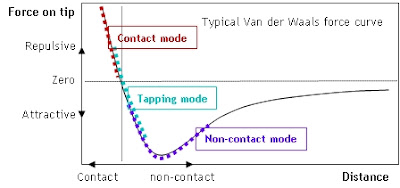Two types 1. Contact force 2. Non-contact force or action at a distance force 1. Contact force 2. Non-contact force Contact Force What is the contact force? Answer – for an object to be pulled or pushed there should be a contract between the two objects Give examples of contact force. pulling a box when a called spring is stretched types of contact force How many types of contact forces are there?Two types 1. Muscular force 2. Frictional force 1. Muscular 2. Frictional Muscular Force What is the muscular force? Answer – The force exerted by muscles of the human or animal body. Give examples of muscular force. Lifting certain objects, walking, running Frictional force What is the frictional force? Answer – The force acting between two surfaces in contact which opposes the motion of one body over the other. Give examples of frictional force? Rolling ball stops after some time due to frictional force. rolling ball stops due to frictional force. Non –contact force What is a non-contact force? Answer – The force which can act even without any actual contact between the two objects is called non-contact force. Which can act without any actual contact Types of non-contact force What are the types of non-contact force?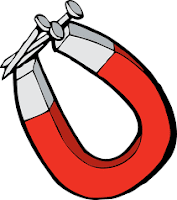1. Magnetic force 2. Electrostatic force 3. Gravitational force · Magnetic · Electrostatic ·Gravitational magnetic force What is the magnetic force? Answer – Force exerted by a magnet. Give examples of the magnetic force? A magnet attracts nails and pins made from iron electrostatic force What is the electrostatic force? Answer – the force exerted by the electrified body Give an example of electrostatic force? A charged scale or comb attracting tiny pieces of paper. gravitational force what is a gravitational force? Answer – the force of attraction between any two objects possessing mass is called gravitational force?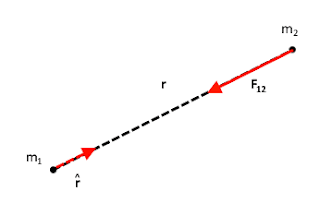Give examples of gravitational force? the ball falls down due to gravitational force. the force of attraction between any two objects

Recapitulation –

In order to revise the topic, the teacher or trainee teacher will ask the following questions.
1. Define force?
2. Name the types of contact and non-contact force.
3. What is the standard unit of force?
4. Which force attracts nails made up of iron from a distance?
Homework –

Fill in the blanks

1. A pull or push acting on a body is called ______
2. SI unit of force is _______
3. Non contact force is also called ________
4. Contact forces area of ______ forces.

Questions –
• Explain contact forces along with their types.
• Explain non-contact forces along with their types?

#### Similar Posts

💁Hello Friends, If You Want To Contribute To Help Other Students To Find All The Stuff At A Single Place, So Feel Free To Send Us Your Notes, Assignments, Study Material, Files, Lesson Plan, Paper, PDF Or PPT Etc. - 👉 Upload Here

अगर आप हमारे पाठकों और अन्य छात्रों की मदद करना चाहते हैं। तो बेझिझक अपने नोट्स, असाइनमेंट, अध्ययन सामग्री, फाइलें, पाठ योजना, पेपर, पीडीएफ या पीपीटी आदि हमें भेज सकते है| -👉Share Now